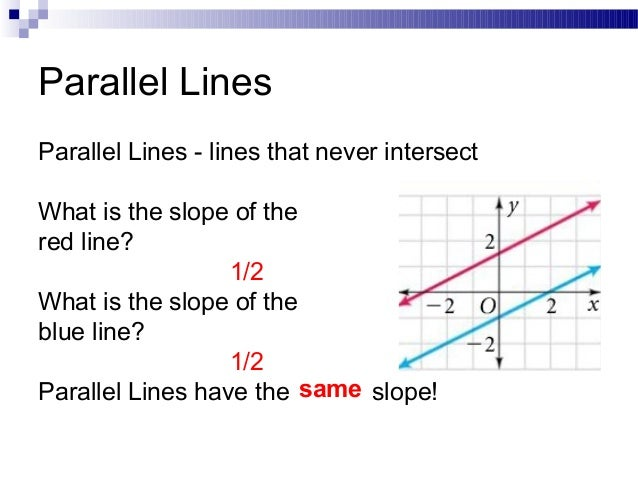# Question #551d4

Dec 8, 2017

5

#### Explanation:

Slope-intercept form is
$y = m x + b$
$m$ = slope
$b$ = y-intercept

The slope of $y = 5 x + 4$
is $5$

And two lines with different slopes will intersect somewhere.
therefore all parallel lines has the same slope.

P.S. If you need help understanding this further please comment

Dec 8, 2017

5

#### Explanation:Essentially, Lines that are parallel means they have the same slope.

So looking at your equation, we see that $y = 5 x + 4$ has a slope of 5, that means the slope of a line that’s parallel to this line will have the same slope, which is also 5.

The slope is labeled as m in an equation in the form of $y = m x + b$. In other words: